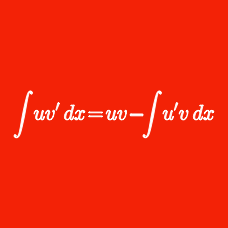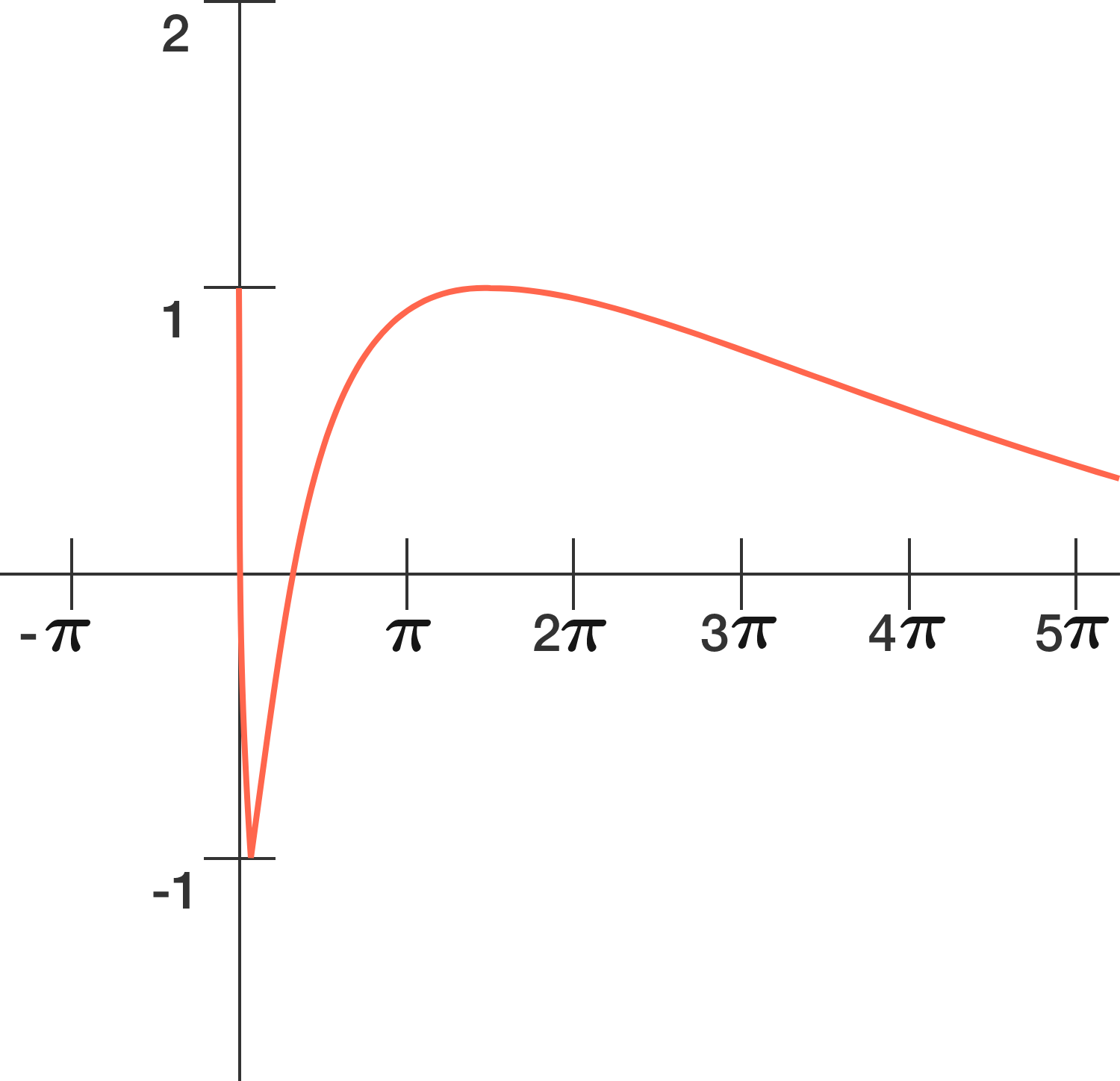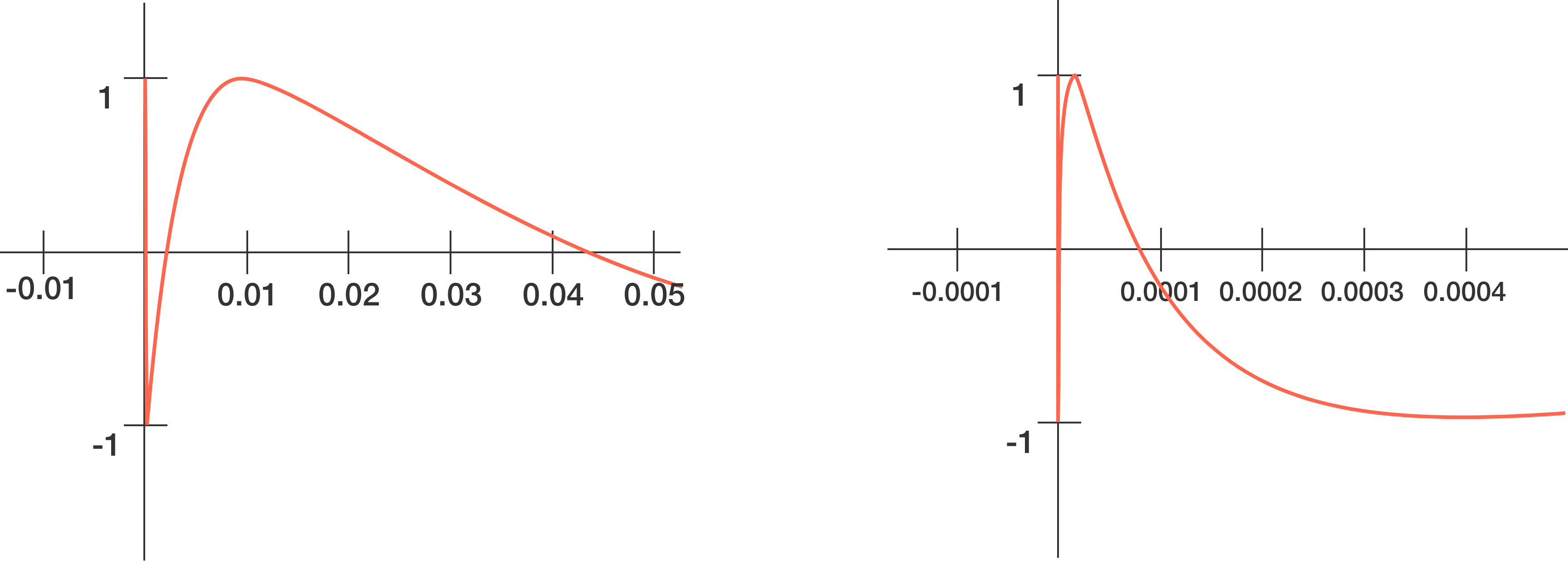Calculus

# Integration Techniques: Level 4 Challenges

$\large \int_{1}^{\infty} \dfrac {2x\{x\} - \{x\}^2} {x^2 \lfloor x \rfloor ^2} \, dx$

If the integral above can be expressed as $$\dfrac{\pi^a - b} c$$, where $$a,b,c$$ are all positive integers, find $$a+b+c$$.

Notations:

$\large \int_0^\infty \frac {\sin x}{xe^x} \, dx = \, ?$

$\large A = \displaystyle\int_{1}^{\infty} \left(\dfrac{1}{u^2} - \dfrac{1}{u^4}\right) \dfrac{du}{\ln u}$

Find the value of $$e^A$$.

Let $$f(x) = x + e^x - 1$$, and let $$f^{-1} (x)$$ denote the inverse function of $$f(x)$$.

Then the integral $$\displaystyle \int_{e}^{1 + e^2} f^{-1} (x) \, dx$$ evaluates to $k_0 + k_1 e^1 + k_2 e^2 + \cdots + k_n e^n ,$ where $$k_0, k_1, \ldots, k_n$$ are rational numbers and $$e\approx 2.718$$ is Euler's number.

If the sum $$k_0 + k_1 + k_2 + \cdots + k_n$$ can be expressed as $$\frac{A}{B},$$ where $$A$$ and $$B$$ are coprime positive integers, what is $$A+B+n?$$The graph of $$f(x) = \sin ( \ln x )$$ (as shown above) looks innocent enough to noticeably oscillate as $$x$$ increases. However, as $$x$$ approaches $$0$$, the oscillations grow rapidly, making $$f(x + \epsilon)$$ vary greatly from $$f(x)$$ around this region, even at very infinitesimal values of $$\epsilon$$.That said, $$f(x)$$ will cross the $$x$$-axis for an infinite number of times from $$x=0$$ to $$x=1$$, creating several regions of the first quadrant enclosed by the curve and the $$x$$-axis.

If the sum of these regions is $$A$$, then determine $$\big\lfloor 10^5 A \big\rfloor$$.

×

Problem Loading...

Note Loading...

Set Loading...